Question

A U-shaped tube open to the air at both ends contains water. A quantity of oil of unknown density is slowly poured into the right arm of the tube until the vertical height of the oil column is 20cm. The top of the oil is 8cm higher than the top of the water. Find the density of the oil.

1.trungdung22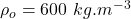is the density of the oil

Explanation:

Given:

• height of oil column,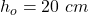• oil column height that is more than the water column height in the other arm,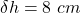Now from the given it is clear that the height of water column is: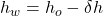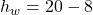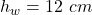Now according to the pressure balance condition of fluid columns:

Pressure due to water column = Pressure due to oil column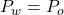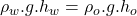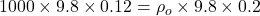is the density of the oil

2.yenoanh

Explanation:

Let the density of oil is d’.

height of water, h = 20 – 8 = 12 cm

height of oil, h’ = 20 cm

density of water, d = 1000 kg/m³

Pressure is balanced

h’ x d’ x g = h x d x g

0.20 x d’ x g = 0.12 x 1000 x g

0.2 d’ = 120

d’ = 600 kg/m³